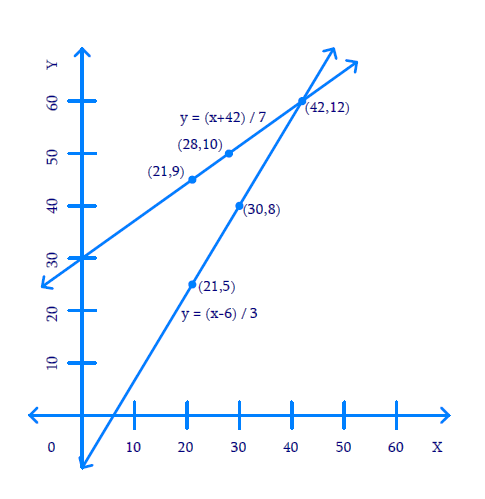# Ex.3.1 Q1 Pair of Linear Equations in Two Variables Solution - NCERT Maths Class 10

Go back to  'Ex.3.1'

## Question

Aftab tells his daughter, “Seven years ago, I was seven times as old as you were then. Also, three years from now, I shall be three times as old as you will be.” (Isn’t this interesting?) Represent this situation algebraically and graphically.

Video Solution
Pair Of Linear Equations In Two Variables
Ex 3.1 | Question 1

## Text Solution

What is Known?

$$7$$ years ago, Aftab was $$7$$ times as old as his daughter then and $$3$$ years from now, Aftab shall be $$3$$ times as old as his daughter will be.

What is Unknown?

Represent the situation algebraically and graphically.

Reasoning:

Assume the present age of Aftab be $$x$$  years and his daughter be $$y$$ years then represent their ages $$7$$ years later and $$3$$ years ago in term of $$x$$ and $$y.$$ Two linear equations can be formed to represent the above situation algebraically.

Using algebraic equation and truth table they can be graphically represented.

Steps:

(i) Present age of Aftab $$= x$$ years and his daughter $$= y$$ years

Therefore, 7 years ago, age of Aftab $$= \left( {x - 7} \right)$$ years and his daughter $$= \left( {y - 7} \right)$$years

Using this information and applying the known condition that $$7$$ years ago, Aftab was $$7$$ times as old as his daughter then:

\begin{align}x - 7 &= 7(y - 7)\\x - 7 &= 7y - 49\\x - 7y - 7 + 49 &= 0\\x - 7y + 42 &= 0\end{align}

After $$3$$ years from now, age of Aftab $$= \left( {x + 3} \right)$$ years and his daughter $$= \left( {y + 3} \right)$$ years and also Aftab will be $$3$$ times as old as his daughter. Then mathematically,

\begin{align}x + 3 &= 3\left( {y + 3} \right)\\x + 3 &= 3y + 9\\x - 3y + 3 - 9 &= 0\\x - 3y - 6 &= 0\end{align}

Algebraic representations, where $$x$$ and $$y$$ are present ages of Aftab and his daughter respectively:

\begin{align}x - 7y + 42 &= 0\qquad(1)\\x - 3y-6 &= 0\qquad(2)\end{align}

Therefore, the algebraic representation is for equation $$1$$ is:

\begin{align}x - 7y + 42 &= 0\\- 7y &= - x - 42\\7y &= x + 42\\y &= \frac{{x + 42}}{7}\end{align}

And, algebraic representation for equation $$(2)$$ is:

\begin{align}x - 3y - 6 &= 0\\- 3y &= - x + 6\\3y &= x - 6\\y &= \frac{{x - 6}}{3}\end{align}

Let us represent these equations graphically. For this, we need at least two solutions for each equation. We give these solutions in table shown below.

For equation $$(1)$$

 $$x$$ $$21$$ $$28$$ $$y = \frac{{x + 42}}{7}$$ $$9$$ $$10$$

For equation (2)

 $$x$$ $$30$$ $$21$$ $$y = \frac{{x - 6}}{3}$$ $$8$$ $$5$$

The graphical representation is as follows.Unit: $$1 \,\rm{cm}=5$$ years.

The answer is $$(42, \;12)$$

Learn from the best math teachers and top your exams

• Live one on one classroom and doubt clearing
• Practice worksheets in and after class for conceptual clarity
• Personalized curriculum to keep up with school